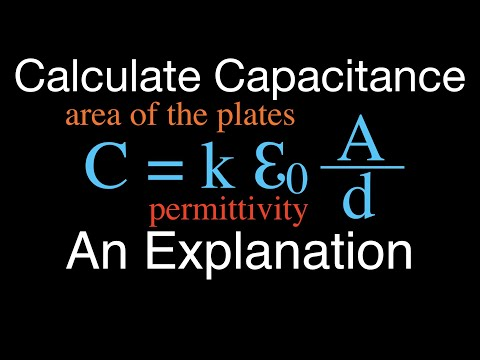# How To Calculate The Capacitance Of A Capacitor

## Video: How To Calculate The Capacitance Of A CapacitorVideo: Capacitors (4 of 9) Calculating the Capacitance of a Capacitor, An Explanation 2023, December

The capacitance of a capacitor depends on the distance between the plates, their area, as well as the relative permittivity of the medium located between them. It is inversely proportional to the first of these parameters and directly proportional to the other two.

## Instructions

### Step 1

Calculate the area of one of the plates (if they are different, then the smaller one), having previously translated the initial data into meters. The calculation method depends on the shape of the plate. For example, for a rectangle: S = ab, where S is the area (m2), a is the length (m), b is the width (m); for a circle: S = π (R ^ 2), where S is the area (m2), π - number "pi", 3, 1415926535 (dimensionless value), R - radius (m). In some capacitors, the plates are rolled up for compactness. Then consider them expanded when calculating.

### Step 2

From the table, the link to which is given at the end of the article, find out the dielectric constant of the medium located between the plates. This is a dimensionless quantity equal to unity for a vacuum. In air, it is so close to unity (1, 00058986) that it is often taken for 1 for simplicity.

### Step 3

Substitute the initial data into the formula: C = (ε abs. Vac. * Ε rel. Things * S) / d, where C is the capacity (F), ε abs. vac. - absolute dielectric constant of vacuum, 8, 8541878176 (F / m), ε rel. real * S is the relative dielectric constant of the substance (dimensionless value), S is the area of the smallest of the plates (m2), d is the distance between the plates (m).

### Step 4

On the capacitors themselves and in the circuits, it is customary to denote capacitance not in farads or millifarads, but, depending on the magnitude, in microfarads, nanofarads and picofarads. Translate the calculation result into those of these units in which it is most convenient to represent it.